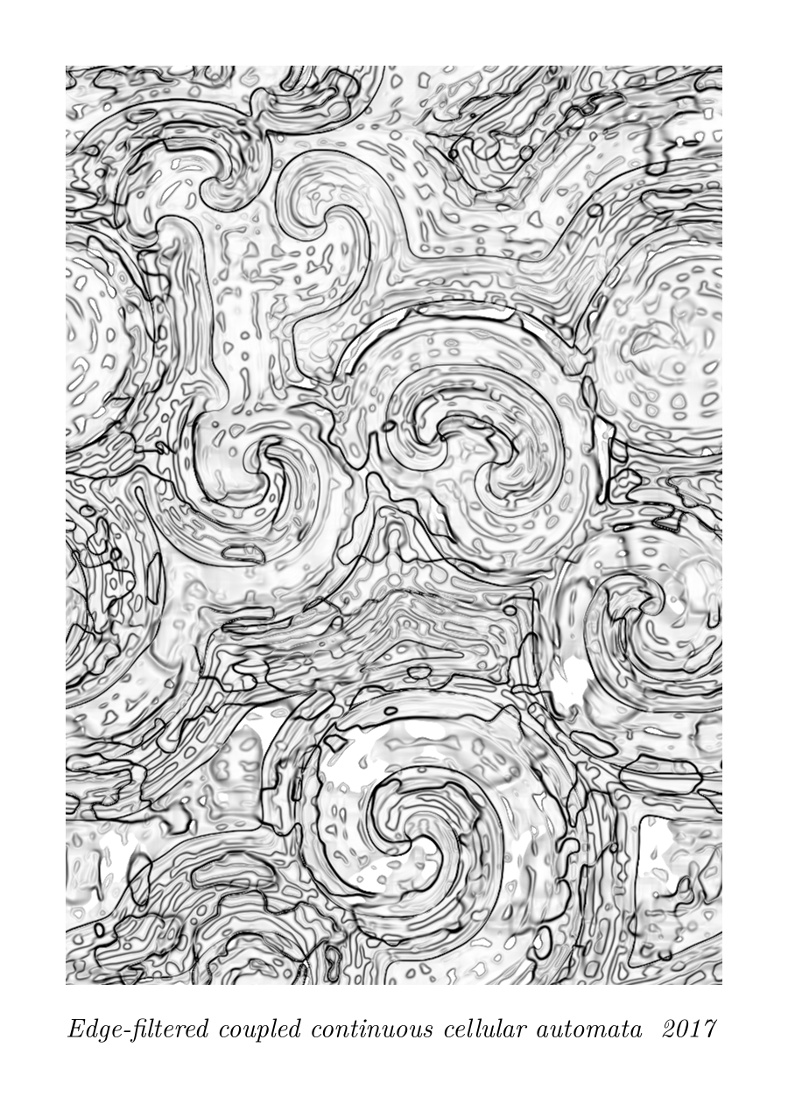# mathr / blog / #

## Coupled continuous cellular automataLate last year I implemented some coupled continuous cellular automata, inspired by Softology's experiments. Now I'm finally getting around to blogging about it. I used OpenGL shaders, here's some of the fragment shader source of the main algorithm:

void main()
{
vec4 s1 = texture(state, coord, 1.0);
vec4 s100 = texture(state, coord, 100.0);
vec4 s;
for (int k = 0; k < 4; ++k)
s[k] = texture(state, coord, blur[k])[k];
vec4 h = texture(history, coord);
s = coupling * (s - s100) + h;
s = speed * s;
s = mix(s1, vec4(0.5) + 0.5 * cos(s), 0.125);
h = mix(s, h, decay);
state_out = s;
history_out = h;
}

The non-linearity of the cos() on the coupled input acts like a "reaction", the blurring (looking up reduced mipmap levels from the texture) acts like "diffusion".

Colouring is done with another affine matrix transform, the output from which is thresholded and clamped, before edge-detection filter is applied. The edge-detection uses dFdx and dFdy, so the results are coarse (these derivatives are typically computed for blocks of 2x2 pixels, rendered together in parallel) - for better results the edge detection could be done in another pass, or the whole thing could run at double the resolution and be resized down to screen size afterwards.

Here's a video of it in action:

Here are some static images:

Here is another video, from January when it was still in colour:

Here's where you can get the code:

git clone https://code.mathr.co.uk/cca.git

Future work might be to do proper Gaussian blurs (it's separable, so even large radius might be feasible in real-time) instead of the cheap (but yielding squarish grid artifacts) mipmap reduction.

EDIT I worked on it some more, now in colour and with a high quality mode that does Gaussian blur (on my system frame rate drops from ~60fps to between ~5fps and ~30fps depending on blur radius). Pictures:

I also added a mutation mode, which randomizes the parameters one by one at random. Here's a final example video showing off the new features: